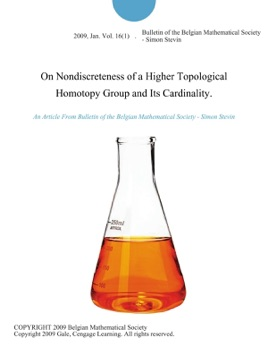• 2,99 €

## Descrizione dell’editore

1 Introduction In 1998, J. Pawlicowski  presented a forcing free proof of a conjecture of Mycielski  that the fundamental group of a connected locally connected compact metric space is either finitely generated or has the power of the continuum. In , Biss equipped the loop space of X with the compact open topology. Then he put a canonical topology on the fundamental group of X as a quotient of Hom(([S.sup.1], 1), (X, x)) which is invariant under the homotopy type of X and denoted it by [[pi].sup.top.sub.n](X, x). He proved among the other things that [[pi].sup.top.sub.n](X, x) is a topological group which is independent of the base point. Recently, P. Fabel  using the Mycielski's conjecture, showed that if X is a peano continuum, then either X has a finitely generated discrete topological fundamental group, or it has a non-discrete topological fundamental group, having the power of the continuum. In , we et al. introduced a topology on higher homotopy groups of a pointed space (X, x) as a quotient of Hom(([I.sup.n], [I.sup.n]), (X, x)) equipped with the compact-open topology and denoted it by [[pi].sup.top.sub.n] (X, x). We proved that [[pi].sup.top.sub.n](X, x) is a topological group. Also, we found necessary and sufficient conditions for which the topology is discrete. In this note, we are going to extend Mycielski's conjecture to higher homotopy groups. At the end, we generalize Fabel's results for topological higher homotopy groups: Suppose X is an (n - 1)-connected locally (n - 1)-connected compact metric space. Then [[pi].sup.top.sub.n](X) is discrete if and only if [[pi].sub.n](X) is finitely generated. Moreover, [[pi].sup.top.sub.n](X) is not discrete if and only if [[pi].sub.n] (X) has the power of the continuum.